# Diamond

The diamond has a circumference of 48cm. Calculate the length of its side in dm.

Result

a =  1.2 dm

#### Solution:Leave us a comment of example and its solution (i.e. if it is still somewhat unclear...):

Showing 0 comments:Be the first to comment!## Next similar examples:

1. Table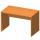Find the circumference of the table, where the long side is 1.28 meters and the short side is 86 cm.
2. Garden plotCalculate how many meters of fence need to fence the square garden with length and width of 22 meters.
3. RectangleCalculate perimeter of the rectangle with sides a=2.4 m and b=1.9 m.
4. RhombusFind the length of each side of rhombus if the perimeter is 49 dm long.
5. Bean bag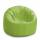A student tossed a bean bag. It landed 216 inches away. How many yards are equal to 216 inches?
6. Zahrada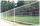Zahrada má rozměry 5m a 400cm. Kolik pletiva třeba na oplocení pozemku?
7. Strip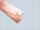From 5.9 cm wide strip should be cut rhombus with area 28 cm2. How long will be its side?
8. HoursThe lesson lasts 45 minutes. For the week, students have 18 lesson hours. How many are the actual hours?
9. Hr to minSue biked to school in 5/12 of an hour. How many minutes did it take her to ride to school?
10. The shopThe shop has 3 hectoliters of water. How many liter bottles is it?
11. Clock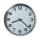How many hours are 15 days?
12. The sum graphically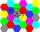Draw a graphically sum of the all sides of 4-gon ABCD.
13. Addition of Roman numbersAdded together and write as decimal number: LXVII + MLXIV
14. Expressions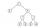Find out value of expressions if a = -1, b =2: x=b - 2a - ab y=a3 - b2 - 2ab z=a2 b3 - a3 b2 w=a + b + a3 - b2
15. If-then equationIf 5x - 17 = -x + 7, then x =
16. Math classificationIn 3A class are 27 students. One-third got a B in math and the rest got A. How many students received a B in math?
17. The resultHow many times I decrease the number 1632 to get the result 24?